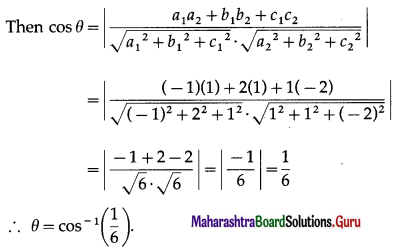Balbharti 12th Maharashtra State Board Maths Solutions Book Pdf Chapter 5 Vectors Ex 5.3 Questions and Answers.

## Maharashtra State Board 12th Maths Solutions Chapter 5 Vectors Ex 5.3

Question 1.
Find two unit vectors each of which is perpendicular to both
$$\bar{u}$$ and $$\bar{v}$$, where $$\bar{u}$$ = $$2 \hat{i}+\hat{j}-2 \hat{k}$$, $$\bar{v}$$ = $$\hat{i}+2 \hat{j}-2 \hat{k}$$
Solution:
Let $$\bar{u}$$ = $$2 \hat{i}+\hat{j}-2 \hat{k}$$
$$\bar{v}$$ = $$\hat{i}+2 \hat{j}-2 \hat{k}$$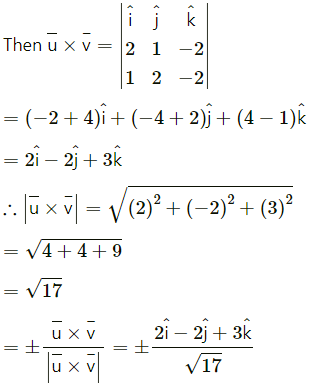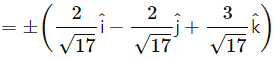Question 2.
If $$\bar{a}$$ and $$\bar{b}$$ are two vectors perpendicular to each other, prove that ($$\bar{a}$$ + $$\bar{b}$$)2 = ($$\bar{a}$$ – $$\bar{b}$$)2
Solution:
$$\bar{a}$$ and $$\bar{b}$$ are perpendicular to each other.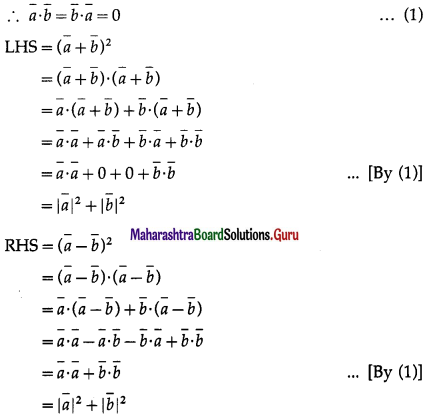∴ LHS = RHS
Hence, ($$\bar{a}$$ + $$\bar{b}$$)2 = ($$\bar{a}$$ – $$\bar{b}$$)2.Question 3.
Find the values of c so that for all real x the vectors $$x c \hat{i}-6 \hat{j}+3 \hat{k}$$ and $$x \hat{i}+2 \hat{j}+2 c x \hat{k}$$ make an obtuse angle.
Solution:
Let $$\bar{a}$$ = $$x c \hat{i}-6 \hat{j}+3 \hat{k}$$ and $$\bar{b}$$ = $$x \hat{i}+2 \hat{j}+2 c x \hat{k}$$
Consider $$\bar{a}$$∙$$\bar{b}$$ = $$(x c \hat{i}-6 \hat{j}+3 \hat{k}) \cdot(x \hat{i}+2 \hat{j}+2 c x \hat{k})$$
= (xc)(x) + (-6)(2) + (3)(2cx)
= cx2 – 12 + 6cx
= cx2 + 6cx – 12
If the angle between $$\bar{a}$$ and $$\bar{b}$$ is obtuse, $$\bar{a}$$∙$$\bar{b}$$ < 0.
∴ cx2 + 6cx – 12 < 0
∴ cx2 + 6cx < 12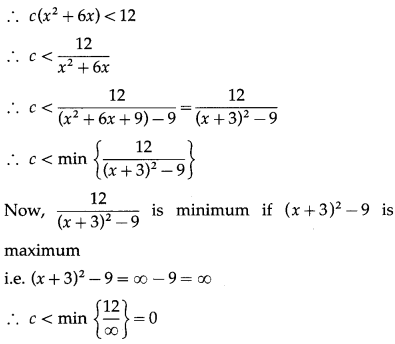∴ c < 0.
Hence, the angle between a and b is obtuse if c < 0.

Question 4.
Show that the sum of the length of projections of $$\hat{p} \hat{i}+q \hat{j}+r \hat{k}$$ on the coordinate axes, where p = 2, q = 3 and r = 4, is 9.
Solution:
Let $$\bar{a}$$ = $$\hat{p} \hat{i}+q \hat{j}+r \hat{k}$$
Projection of $$\bar{a}$$ on X-axis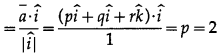Similarly, projections of $$\bar{a}$$ on Y- and Z-axes are 3 and 4 respectively.
∴ sum of these projections = 2 + 3 + 4 = 9.Question 5.
Suppose that all sides of a quadrilateral are equal in length and opposite sides are parallel. Use vector methods to show that the diagonals are perpendicular.
Solution: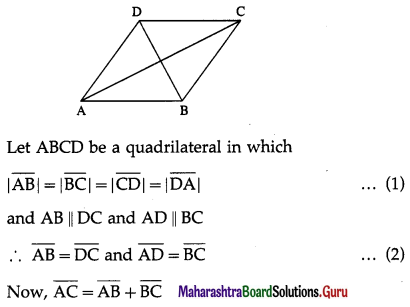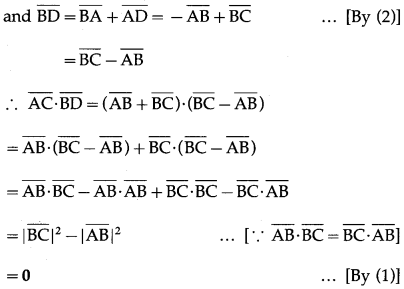∵ $$\overline{\mathrm{AC}}$$, $$\overline{\mathrm{BD}}$$ are non-zero vectors
∴ $$\overline{\mathrm{AC}}$$ is perpendicular to $$\overline{\mathrm{BD}}$$
Hence, the diagonals are perpendicular.

Question 6.
Determine whether $$\bar{a}$$ and $$\bar{b}$$ are orthogonal, parallel or neither.
(i) $$\bar{a}$$ = $$-9 \hat{i}+6 \hat{j}+15 \hat{k}$$, $$\bar{b}$$ = $$6 \hat{i}-4 \hat{j}-10 \hat{k}$$
Solution:
$$\bar{a}$$ = $$-9 \hat{i}+6 \hat{j}+15 \hat{k}$$ = -3$$(3 \hat{i}-2 \hat{j}-5 \hat{k})$$
= $$-\frac{3}{2}(6 \hat{i}-4 \widehat{j}-19 \hat{k})$$
∴ $$\bar{a}$$ = $$-\frac{3}{2} \bar{b}$$
i.e. $$\bar{a}$$ is a non-zero scalar multiple of $$\bar{b}$$
Hence, $$\bar{a}$$ is parallel to $$\bar{b}$$.(ii) $$\bar{a}$$ = $$2 \hat{i}+3 \hat{j}-\hat{k}$$, $$\bar{b}$$ = $$5 \hat{i}-2 \hat{j}+4 \hat{k}$$
Solution:
$$\bar{a} \cdot \bar{b}$$ = $$(2 \hat{i}+3 \hat{j}-\hat{k}) \cdot(5 \hat{i}-2 \hat{j}+4 \hat{k})$$
= (2)(5) + (3)(-2) + (-1)(4)
= 10 – 6 – 4 = 0
Since, $$\bar{a}$$, $$\bar{b}$$ are non-zero vectors and $$\bar{a} \cdot \bar{b}$$ = 0, $$\bar{a}$$ is orthogonal to $$\bar{b}$$.

(iii) $$\bar{a}$$ = $$-\frac{3}{5} \hat{i}+\frac{1}{2} \hat{j}+\frac{1}{3} \hat{k}$$, $$\bar{b}$$ = $$5 \hat{i}+4 \hat{j}+3 \hat{k}$$.
Solution: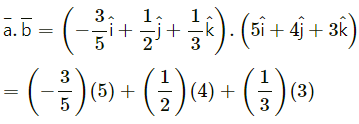= -3 + 2 + 1
= 0
Since, $$\bar{a}$$, $$\bar{b}$$ are non-zero vectors and $$\bar{a} \cdot \bar{b}$$ = 0
$$\bar{a}$$ is orthogonal to $$\bar{b}$$.

(iv) $$\bar{a}$$ = $$4 \hat{i}-\hat{j}+6 \hat{k}$$, $$\bar{a}$$ = $$5 \hat{i}-2 \hat{j}+4 \hat{k}$$
Solution:
$$\bar{a} \cdot \bar{b}$$ = $$(4 \hat{i}-\hat{j}+6 \hat{k}) \cdot(5 \hat{i}-2 \hat{j}+4 \hat{k})$$
= (4)(5) + (-1)(-2) + (6)(4)
= 20 + 2 + 24
= 46 ≠ 0
∴ $$\bar{a}$$ is not orthogonal to $$\bar{b}$$.
It is clear that $$\bar{a}$$ is not a scalar multiple of $$\bar{b}$$.
∴ $$\bar{a}$$ is not parallel to $$\bar{b}$$.
Hence, $$\bar{a}$$ is neither parallel nor orthogonal to $$\bar{b}$$.Question 7.
Find the angle P of the triangle whose vertices are P(0, -1, -2), Q(3, 1, 4) and R(5, 7, 1).
Solution:
The position vectors $$\bar{p}$$, $$\bar{q}$$ and $$\bar{r}$$ of the points P(0, -1, -2), Q(3, 1, 4) and R(5, 7, 1) are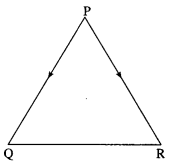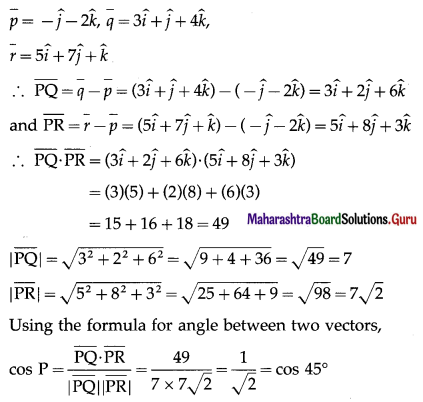∴ P = 45°

Question 8.
If$$\hat{p}$$, $$\hat{q}$$, and $$\hat{r}$$ are unit vectors, find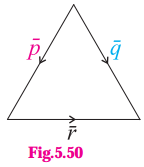(i) $$\hat{p} \cdot \hat{q}$$
Solution: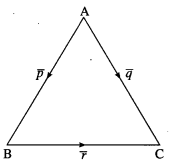Let the triangle be denoted by ABC,
where $$\overline{\mathrm{AB}}$$ = $$\bar{p}$$, $$\overline{\mathrm{AC}}$$ = $$\bar{q}$$ and $$\overline{\mathrm{BC}}$$ = $$\bar{r}$$
∵ $$\bar{p}$$, $$\bar{r}$$, $$\bar{r}$$ are unit vectors.
∴ l(AB) = l(BC) = l(CA) = 1
∴ the triangle is equilateral
∴ ∠A = ∠B = ∠C = 60°
(i) Using the formula for angle between two vectors,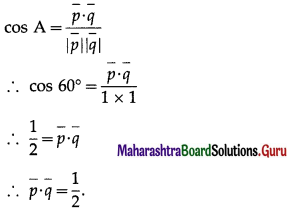(ii) $$\hat{p} \cdot \hat{r}$$
Solution: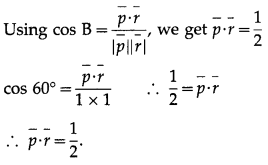Question 9.
Prove by vector method that the angle subtended on semicircle is a right angle.
Solution:
Let seg AB be a diameter of a circle with centre C and P be any point on the circle other than A and B. Then ∠APB is an angle subtended on a semicircle.
Let $$\overline{\mathrm{AC}}$$ = $$\overline{\mathrm{CB}}$$ = $$\bar{a}$$ and $$\overline{\mathrm{CP}}$$ = $$\bar{r}$$.
Then|$$\bar{a}$$| = |$$\bar{r}$$| …(1)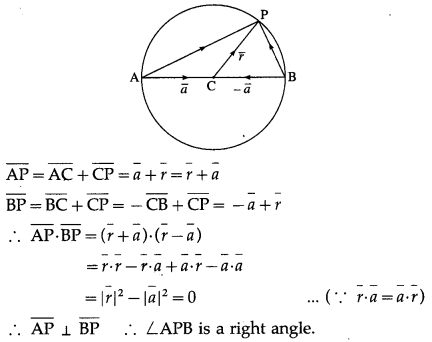Hence, the angle subtended on a semicircle is the right angle.

Question 10.
If a vector has direction angles 45ºand 60º find the third direction angle.
Solution:
Let α = 45°, β = 60°
We have to find γ.
∴ cos2α + cos2β + cos2γ = 1
∴ cos245° + cos260° + cos2γ = 1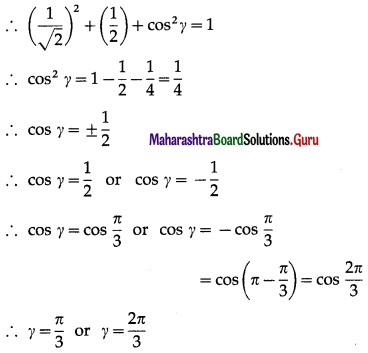Hence, the third direction angle is $$\frac{\pi}{3}$$ or $$\frac{2 \pi}{3}$$.Question 11.
If a line makes angles 90º, 135º, 45º with the X, Y and Z axes respectively, then find its direction cosines.
Solution:
Let l, m, n be the direction cosines of the line.
Then l = cos α, m = cos β, n = cos γ
Here, α = 90°, β = 135° and γ = 45°
∴ l = cos 90° = 0
m = cos 135° = cos (180° – 45°) = -cos 45° = $$-\frac{1}{\sqrt{2}}$$ and n = cos 45° = $$\frac{1}{\sqrt{2}}$$
∴ the direction cosines of the line are 0, $$-\frac{1}{\sqrt{2}}, \frac{1}{\sqrt{2}}$$.

Question 12.
If a line has the direction ratios, 4, -12, 18 then find its direction cosines.
Solution:
The direction ratios of the line are a = 4, b = -12, c = 18.
Let l, m, n be the direction cosines of the line.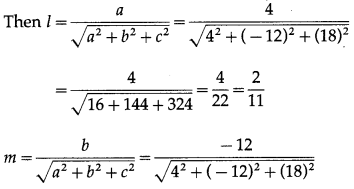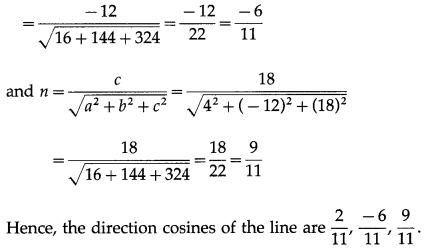Question 13.
The direction ratios of $$\overline{A B}$$ are -2, 2, 1. If A = (4, 1, 5) and l(AB) = 6 units, find B.
Solution:
The direction ratio of $$\overline{A B}$$ are -2, 2, 1.
∴ the direction cosines of $$\overline{A B}$$ are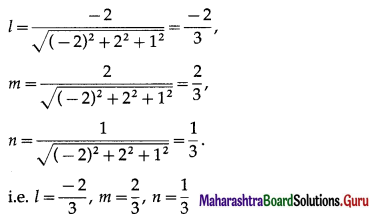The coordinates of the points which are at a distance of d units from the point (x1, y1, z1) are given by (x1 ± ld, y1 ± md, z1 ± nd)
Here x1 = 4, y1 = 1, z1 = 5, d = 6, l = $$-\frac{2}{3}$$, m = $$\frac{2}{3}$$, n = $$\frac{1}{3}$$
∴ the coordinates of the requited points are
(4 ± $$\left(-\frac{2}{3}\right)$$6, 1 ± $$\frac{2}{3}$$(6), 5 ± $$\frac{1}{3}$$(6))
i.e. (4 – 4, 1 + 4, 5 + 2) and (4 + 4, 1 – 4, 5 – 2)
i.e. (0, 5, 7) and (8, -3, 3).

Question 14.
Find the angle between the lines whose direction cosines l, m, n satisfy the equations 5l + m + 3n = 0 and 5mn – 2nl + 6lm = 0.
Solution:
Given, 5l + m + 3n = 0 …(1)
and 5mn – 2nl + 6lm = 0 …(2)
From (1), m = -(51 + 3n)
Putting the value of m in equation (2), we get,
-5(5l + 3n)n – 2nl – 6l(5l + 3n) = 0
∴ -25ln – 15n2 – 2nl – 30l2 – 18ln = 0
∴ – 30l2 – 45ln – 15n2 = 0
∴ 2l2 + 3ln + n2 = 0
∴ 2l2 + 2ln + ln + n2 = 0
∴ 2l(l + n) + n(l + n) = 0
∴ (l + n)(2l + n) = 0
∴ l + n = 0 or 2l + n = 0
l = -n or n = -2l
Now, m = -(5l + 3n), therefore, if l = -n,
m = -(-5n + 3n) = 2n
∴ -l = $$\frac{m}{2}$$ = n
∴ $$\frac{l}{-1}=\frac{m}{2}=\frac{n}{1}$$
∴ the direction ratios of the first line are
a1 = -1, b1 = 2, c1 = 1
If n = -2l, m = -(5l – 6l) – l
∴ l = m = $$\frac{n}{-2}$$
∴ $$\frac{l}{1}=\frac{m}{1}=\frac{n}{-2}$$
∴ the direction ratios of the second line are
a2 = -1, b2 = 1, c2 = -2
Let θ be the angle between the lines.